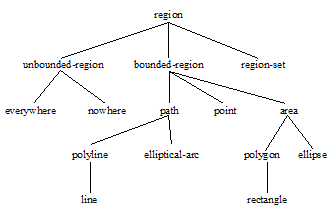### 2.5.1 Regions in CLIM

A region is an object that denotes a set of points in the plane. Regions include their boundaries; that is, they are closed. Regions have infinite resolution.

A bounded region is a region that contains at least one point for which there exists a number, d , called the region's diameter, such that if p1 and p2 are points in the region, the distance between p1 and p2 is always less than or equal to d .

An unbounded region either contains no points or contains points arbitrarily far apart. +nowhere+ and +everywhere+ are examples of unbounded regions.

Another way to describe a region is to say that it maps every ( x, y ) pair into either true or false (meaning member or not a member, respectively, of the region). Later, in Chapter 5, we will generalize a region to something called an ink that maps every point ( x, y ) into color and opacity values.

CLIM classifies the various types of regions in the following way. All regions are a subclass of region , and all bounded regions are also a subclass of either point , path , or area , as shown in Figure 9..###### Figure 9. The Class Structure for All Regions
`region [Protocol Class]	`

Summary: The protocol class that corresponds to a set of points. This includes both bounded and unbounded regions. This is a subclass of ink (see Chapter 5, Drawing in Color for details).

If you want to create a new class that behaves like a region, it should be a subclass of region. Subclasses of region must obey the region protocol.

There is no general constructor called make-region because of the impossibility of a uniform way to specify the arguments to such a function.

`regionp [Function]	`

Arguments: object

Summary: Returns t if object is a region; otherwise, it returns nil .

`path [Protocol Class]	`

Summary: The protocol class path denotes bounded regions that have dimensionality 1 (that is, lines or curves). It is a subclass of region and bounding-rectangle . If you want to create a new class that behaves like a path, it should be a subclass of path. Subclasses of path must obey the path protocol.

Constructing a path object with no length (via make-line* , for example) canonicalizes it to +nowhere+ .

Some rendering models support the constructing of areas by filling a closed path. In this case, the path needs a direction associated with it. Since CLIM does not currently support the path-filling model, paths are directionless.

`pathp [Function]	`

Arguments: object

Summary: Returns t if object is a path; otherwise, it returns nil .

Note that constructing a path object with no length (by calling make-line with two coincident points, for example) canonicalizes it to +nowhere+ .

`area [Protocol Class]	`

Summary: The protocol class area denotes bounded regions that have dimensionality 2 (that is, are flat surfaces). It is a subclass of region and bounding-rectangle . If you want to create a new class that behaves like an area, it should be a subclass of area . Subclasses of area must obey the area protocol.

Note that constructing an area object with no area (by calling make-rectangle with two coincident points, for example) canonicalizes it to +nowhere+ .

`areap [Function]	`

Arguments: object

Summary: Returns t if object is an area; otherwise, it returns nil .

`coordinate `

Summary: The type that represents a coordinate. All of the specific region classes and subclasses of bounding-rectangle will use this type to store their coordinates. However, the constructor functions for the region classes and for bounding rectangles accept numbers of any type and coerce them to coordinate .

The following two constants represent the regions that correspond, respectively, to all of the points on the drawing plane and to none of the points on the drawing plane.

+everywhere+

Summary: The region that includes all the points on the infinite drawing plane.

+nowhere+

Summary: The empty region (the opposite of +everywhere+ ).

#### 2.5.1.2 Composition of CLIM Regions

Common Lisp Interface Manager 2.0 User's Guide - 7 Aug 2017